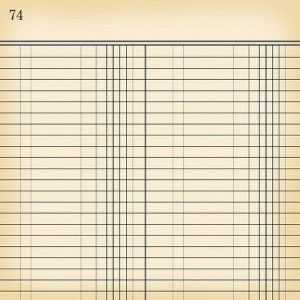# Ledger Paper Size

The term ‘ledger’ refers to the traditional size of paper commonlyused in North American countries such as Canada and the U.S. The other available sizes under this kind of system are the tabloid, legal, letter as well as the junior legal. In order to differentiate one particular size from the other, it is good to know the ledger paper size.

### The Size of a Ledger Paper

The size of a ledger paper is 432 by 279 millimeters or 17 by 11 inches. Using the North American system, it is the biggest amongst the different paper sizes. After that comes the tabloid, a size with dimensions of 279 by 432 millimeters or 11 by 17 inches. The next one is the legal size, which measures 216 by 356 millimeters or 8.5 by 14 inches. The letter size measures 216 by 279 millimeters or 8.5 by 11 inches. The smallest size of paper is the junior legal, which only measures 203 by 127 millimeters or 8 by 5 inches.

### Additional Facts and Other Interesting Details

In addition to the North American system of sizing papers, the ANSI paper sizes are also available. Adopted by the American National Standards Institute, it classifies the size of paper into five different types, namely ANSI A, ANSI B, ANSI C, ANSI D and ANSI E.

The ANSI A is equivalent to the A4 size of the ISO paper format, the measurement of which is 216 by 279 millimeters or 8.5 by 11 inches. The ANSI B is the same as the A3 size of the ISO format. It measures 432 by 279 millimeters or 17 by 11 inches. The size of the ANSI C is the same as the size A2 of the ISO paper sizes, the measurement of which is 432 by 559 millimeters or 17 by 22 inches.

The size of the ANSI D is similar to the ISO paper size A1, the dimensions of which are 559 by 864 millimeters or 22 by 34 inches. Lastly, there is the ANSI E, the equivalent of which is the A0 of the ISO sizes. Its dimensions are 864 by 1,118 millimeters or 34 by 44 inches.

The papers used in developing photographs follow a different format. For instance, the dimensions of a 2R are 64 by 89 millimeters or 2.5 by 3.5 inches. The 3R paper measures 89 by 127 millimeters or 3.5 by 5 inches. The 5R paper is bigger than these two sizes, the dimensions of which are 127 by 178 millimeters or 5 by 7 inches. The 11R is the biggest paper size for photographs, which measures 279 by 356 millimeters or 11 by 14 inches.